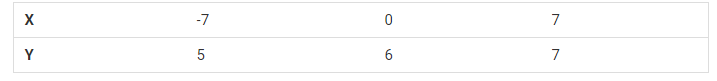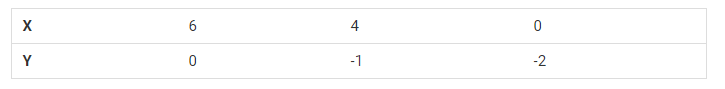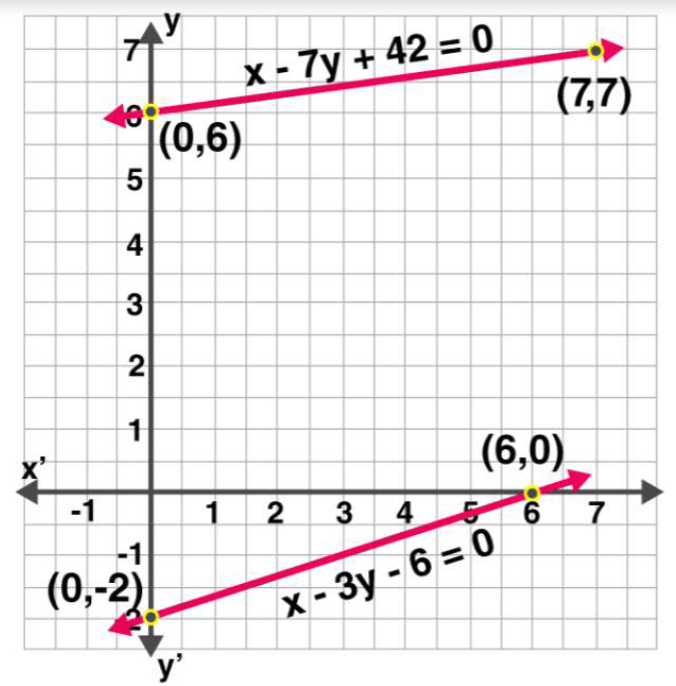Guru

# Aftab tells his daughter, “Seven years ago, I was seven times as old as you were then. Also, three years from now, I shall be three times as old as you will be.” (Isn’t this interesting?) Represent this situation algebraically and graphically. Q.1

• 0

I am finding some problem on solving this question. Its time taking question. So i want best option for solving this question. Aftab tells his daughter, “Seven years ago, I was seven times as old as you were then. Also, three years from now, I shall be three times as old as you will be.” (Isn’t this interesting?) Represent this situation algebraically and graphically.

Share

1. Let the present age of Aftab be ‘x’.

And, the present age of his daughter be ‘y’.

Now, we can write, seven years ago,

Age of Aftab = x-7

Age of his daughter = y-7

According to the question,

x−7 = 7(y−7)

⇒x−7 = 7y−49

⇒x−7y = −42         ………………………(i)

Also, three years from now or after three years,

Age of Aftab will become = x+3.

Age of his daughter will become = y+3

According to the situation given,

x+3 = 3(y+3)

⇒x+3 = 3y+9

⇒x−3y = 6       …………..…………………(ii)

Subtracting equation (i) from equation (ii) we have

(x−3y)−(x−7y) = 6−(−42)

⇒−3y+7y = 6+42

⇒4y = 48

⇒y = 12

The algebraic equation is represented by

x−7y = −42

x−3y = 6

For, x−7y = −42 or x = −42+7y

The solution table isFor,  x−3y = 6   or     x = 6+3y

The solution table isThe graphical representation is:• 0